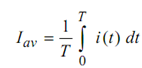## Average Value Assignment Help

Assignment Help: >> RMS and Average Value - Average Value

Average Value:

The average value of alternating current is described by that steady value of current which transfers the same amount of charge across any given circuit as could be transferred by the alternating current for the same time.Note : Waveforms with half wave symmetry, i.e.

f (t ) =  f ( t +( ½) T )

where  T = time period.

Then the average value of such waves for the complete time period is zero (because positive and negative halves are equal in one time period). For such waves, the average value is calculated over the positive half of the period. It is sometimes called the half cycle average. (All the waveforms of Figure have such symmetry).